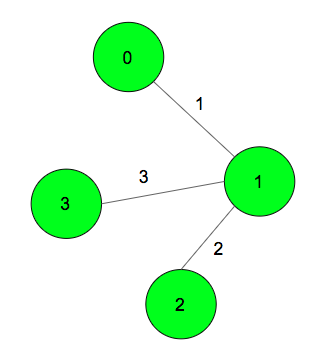# Minimum cost path from source node to destination node via an intermediate node

Given an undirected weighted graph. The task is to find the minimum cost of the path from source node to the destination node via an intermediate node.

Note: If an edge is traveled twice, only once weight is calculated as cost.Examples:

Input: source = 0, destination = 2, intermediate = 3;
Output: 6
The minimum cost path 0->1->3->1->2
The edge (1-3) occurs twice in the path, but its weight is

Input: source = 0, destination = 2, intermediate = 1;
Output: 3
The minimum cost path is 0->1>2

## Recommended: Please try your approach on {IDE} first, before moving on to the solution.

Approach: Let suppose take a path P1 from Source to intermediate, and a path P2 from intermediate to destination. There can be some common edges among these 2 paths. Hence, the optimal path will always have the following form: for any node U, the walk consists of edges on the shortest path from Source to U, from intermediate to U, and from destination to U. Hence, if dist(a, b) is the cost of shortest path between node a and b, the required minimum cost path will be min{ dist(Source, U) + dist(intermediate, U) + dist(destination, U) } for all U. The Minimum distance of all nodes from Source, intermediate, and destination can be found by doing Dijkstra’s Shortest Path algorithm from these 3 nodes.

Below is the implementation of the above approach.

 `// CPP program to find minimum distance between ` `// source and destination node and visiting ` `// of intermediate node is compulsory ` `#include ` `using` `namespace` `std; ` `#define MAXN 100005 ` ` `  `// to strore maped values of graph ` `vector > v[MAXN]; ` ` `  `// to store distance of ` `// all nodes from the source node ` `int` `dist[MAXN]; ` ` `  `// Dijkstra's algorithm to find ` `// shortest path from source to node ` `void` `dijkstra(``int` `source, ``int` `n) ` `{ ` `    ``// set all the vertices ` `    ``// distances as infinity ` `    ``for` `(``int` `i = 0; i < n; i++) ` `        ``dist[i] = INT_MAX; ` ` `  `    ``// set all vertex as unvisited ` `    ``bool` `vis[n]; ` `    ``memset``(vis, ``false``, ``sizeof` `vis); ` ` `  `    ``// make distance from source ` `    ``// vertex to source vertex is zero ` `    ``dist = 0; ` ` `  `    ``// // multiset do the job ` `    ``// as a min-priority queue ` `    ``multiset > s; ` ` `  `    ``// insert the source node with distance = 0 ` `    ``s.insert({ 0, source }); ` ` `  `    ``while` `(!s.empty()) { ` `        ``pair<``int``, ``int``> p = *s.begin(); ` `        ``// pop the vertex with the minimum distance ` `        ``s.erase(s.begin()); ` ` `  `        ``int` `x = p.second; ` `        ``int` `wei = p.first; ` ` `  `        ``// check if the popped vertex ` `        ``// is visited before ` `        ``if` `(vis[x]) ` `            ``continue``; ` ` `  `        ``vis[x] = ``true``; ` ` `  `        ``for` `(``int` `i = 0; i < v[x].size(); i++) { ` `            ``int` `e = v[x][i].first; ` `            ``int` `w = v[x][i].second; ` ` `  `            ``// check if the next vertex ` `            ``// distance could be minimized ` `            ``if` `(dist[x] + w < dist[e]) { ` ` `  `                ``dist[e] = dist[x] + w; ` ` `  `                ``// insert the next vertex ` `                ``// with the updated distance ` `                ``s.insert({ dist[e], e }); ` `            ``} ` `        ``} ` `    ``} ` `} ` ` `  `// function to add edges in graph ` `void` `add_edge(``int` `s, ``int` `t, ``int` `weight) ` `{ ` `    ``v[s].push_back({ t, weight }); ` `    ``v[t].push_back({ s, weight }); ` `} ` ` `  `// function to find the minimum shortest path ` `int` `solve(``int` `source, ``int` `destination,  ` `               ``int` `intermediate, ``int` `n) ` `{ ` `    ``int` `ans = INT_MAX; ` ` `  `    ``dijkstra(source, n); ` ` `  `    ``// store distance from source to ` `    ``// all other vertices ` `    ``int` `dsource[n]; ` `    ``for` `(``int` `i = 0; i < n; i++) ` `        ``dsource[i] = dist[i]; ` ` `  `    ``dijkstra(destination, n); ` `    ``// store distance from destination ` `    ``// to all other vertices ` `    ``int` `ddestination[n]; ` `    ``for` `(``int` `i = 0; i < n; i++) ` `        ``ddestination[i] = dist[i]; ` ` `  `    ``dijkstra(intermediate, n); ` `    ``// store distance from intermediate ` `    ``// to all other vertices ` `    ``int` `dintermediate[n]; ` `    ``for` `(``int` `i = 0; i < n; i++) ` `        ``dintermediate[i] = dist[i]; ` ` `  `    ``// find required answer ` `    ``for` `(``int` `i = 0; i < n; i++) ` `        ``ans = min(ans, dsource[i] + ddestination[i] ` `                                  ``+ dintermediate[i]); ` ` `  `    ``return` `ans; ` `} ` ` `  `// Driver code ` `int` `main() ` `{ ` ` `  `    ``int` `n = 4; ` `    ``int` `source = 0, destination = 2, intermediate = 3; ` ` `  `    ``// add edges in graph ` `    ``add_edge(0, 1, 1); ` `    ``add_edge(1, 2, 2); ` `    ``add_edge(1, 3, 3); ` ` `  `    ``// function call for minimum shortest path ` `    ``cout << solve(source, destination, intermediate, n); ` ` `  `    ``return` `0; ` `} `

Output:

```6
```

Time complexity: O((N + M) * logN), where N is number of nodes, M is number of edges.
Auxiliary Space: O(N+M)

My Personal Notes arrow_drop_uppawanasipugmailcom

If you like GeeksforGeeks and would like to contribute, you can also write an article using contribute.geeksforgeeks.org or mail your article to contribute@geeksforgeeks.org. See your article appearing on the GeeksforGeeks main page and help other Geeks.

Please Improve this article if you find anything incorrect by clicking on the "Improve Article" button below.

Article Tags :
Practice Tags :

Be the First to upvote.

Please write to us at contribute@geeksforgeeks.org to report any issue with the above content.